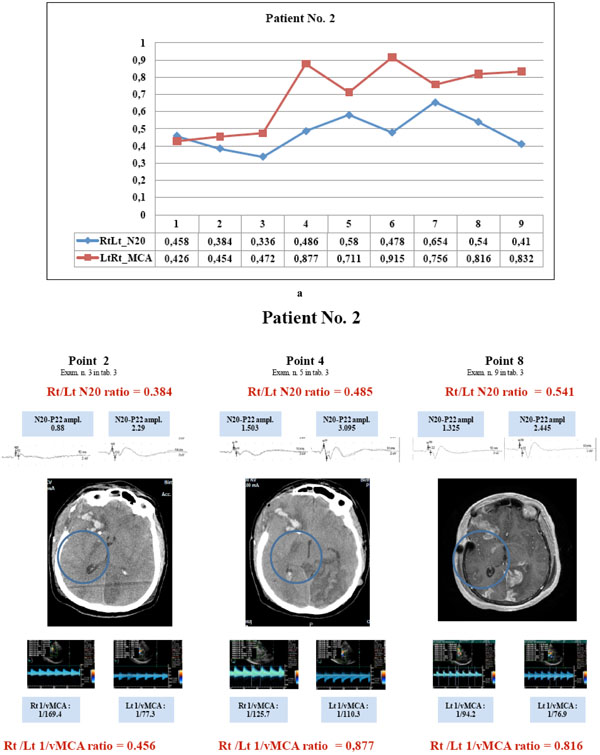Fig. (10) Patient No. 2 showed parallel courses of very low N20 and 1/v MCA ratio (<0.65) detected in the first three examinations (a, points 1to 3), corresponding with CT image of hypodesity of the right capsula (b, point 2); in the subsequent examinations, the divergent courses of N20 (< 0.65) and 1/vMCA (>0.65) and non correlated ratios (p= 0.2 r = 0.46, C.I. for r : -0.28 to 0.86) corresponded with CT and MRI images of structural infarct in the same area (b, point 4 and 8). Right N20 amplitude was < 1.2µV at points 1to 3 and 6 to7 (see examinations 2,3,4,7,8 in Table 3) and =< 1.5 µV at points 4,5, 8and 9 (see examinations 5,6,9,10 in Table 3); right MCA vasospasm (BFV >120 <180 cm/sec) was detected at points 1to 6. Clinical hemiparesis was always evident. (N20 amplitude is expressed in µV and MCA blood flow velocity in cm/sec).